# How to Calculate Percentages?

Percentages are a versatile mathematical instrument to express parts of a whole, where the whole is always 100. Schools, college students, and people at work use them all the time.

But they aren't just for professional reasons. Knowing percentages well can help you determine if the service charge on your restaurant bill is correct, what percentage you should pay in taxes, or what percent of your income is left.

This relationship or fraction/ratio between a part of something and the whole multiplied by 100 is called a percentage (%). Calculating percentages is always handy, especially if you don't have a calculator on hand.

You can do this in a few simple methods, which is what this article will delineate. In this instance, we use the unitary method. However, we will also provide an alternative wherein the denominator is converted to a hundred.

## Method 1: Converting Decimals into Percentages

A decimal is easily converted into a percentage by multiplying it by 100. For example, 0.45 as a decimal is merely 45/100 or 45%.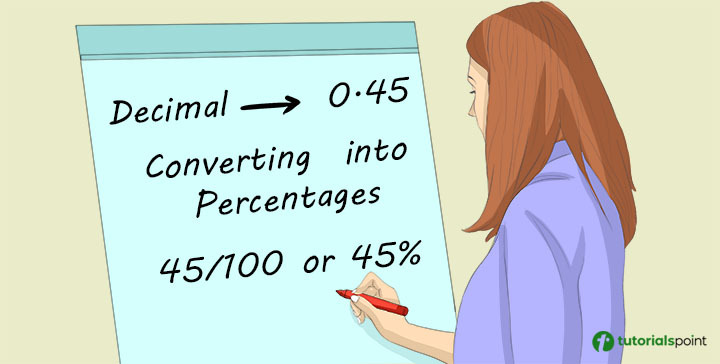If you get a fraction, like 9/20, the decimal value of 0.45 has to be calculated by dividing the numerator by the denominator and multiplying the answer by 100.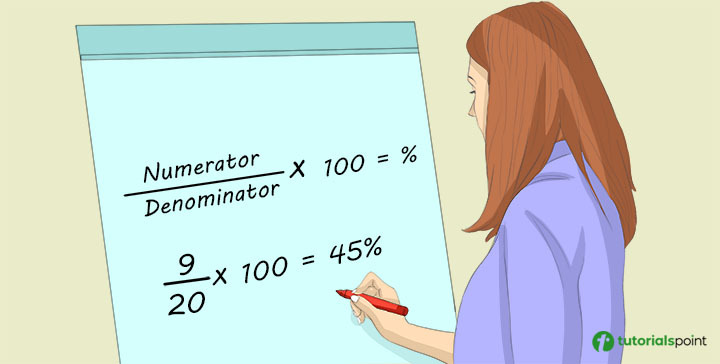## Method 2: Converting Fractions into Percentages

If you have a readymade fraction like 9/20, you must multiply the fraction by 100 to find the percentage, i.e., 45%. Mostly the fractions are proper fractions, in which the numerator is smaller and the denominator is more significant.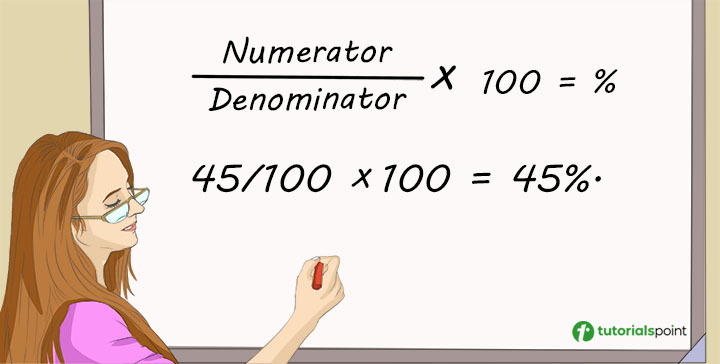Follow this method if you are given a situation where you are asked to calculate a percentage from shared values. Using the same number, suppose there is a bag of 20 chocolates. Of these, nine chocolates are eaten. What percentage of chocolates were consumed?

The part of the whole, i.e., the part is eaten, is taken as the numerator, while the whole is taken as the denominator. Hence, 9/20 *100 = 45%.

Alternatively, you could convert the denominator into 100 if that's easier for you to calculate. So, if you convert 20 into 100 by multiplying it by 5, the numerator, i.e., nine, should also be multiplied by 5. Hence it becomes 45/100 *100, which is 45%.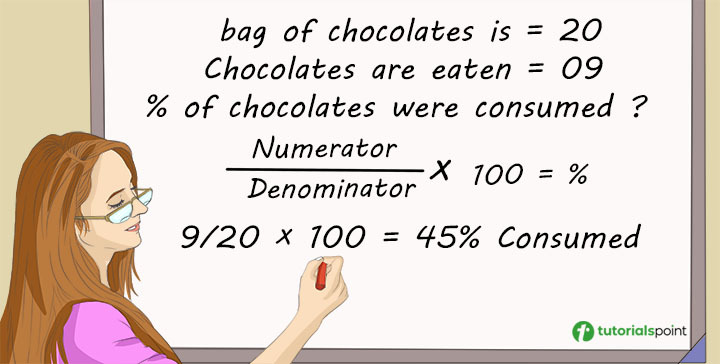The standard formula to calculate percentage is - partial value (to be expressed as a percentage)/ total value *100

## Method 3: Calculating Percentage Increase and Decrease

To calculate any increase or decrease of a whole number/value in terms of percentage, you can use the following formulas −

For percentage increase: (New value – Original Value), i.e., the difference increase/ Original Value *100.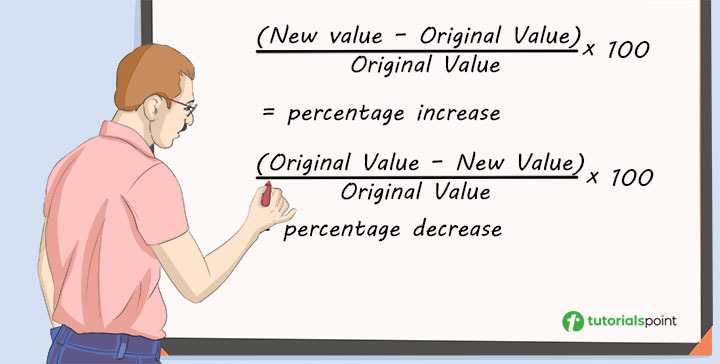For percentage decrease: (Original Value – New Value)/Original Value *100

In essence, the difference between the two values substitutes the partial value as the numerator.

## Method 4: Using Percentage of a Whole to Find the Partial Value

This is a simple method. Suppose you are asked to find 4% of 200 barrels of wine. Place 3 as the numerator and 100 as the denominator, i.e., 4/100, and multiply by the total amount, i.e., 200 barrels of wine.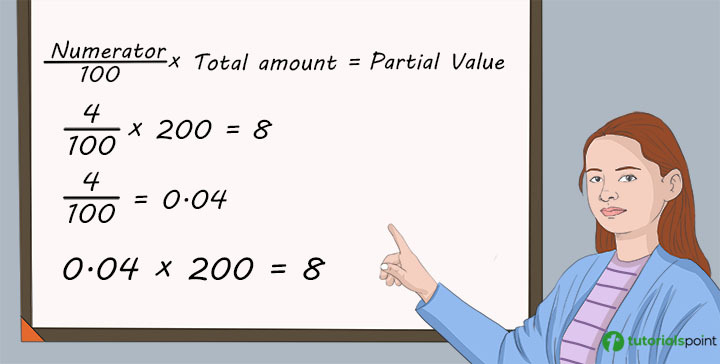So, 4/100* 200 would yield 8 barrels of wine. Alternatively, you can convert the percentage into a decimal, i.e., 4/100 is 0.04. Then 0.04 multiplied by 200 also yield the same outcome – 8 barrels of wine. This is also called the backward or reverse percentage method, which allows you to calculate a value based on a percentage and a whole number, yielding the value the percentage represents.

The formula for this is as follows: Percentage(P)/100= Part Value/Whole Value. For example, if we say taxes have risen to 5% of a $150 meal, how much would the tax amount be? i.e., 5/100 = Z/1500 or 0.05 = Z/15, which can be cross-multiplied to get$7.5 as taxes.

This can also be expressed as X of Y Equals Z, where X is the percentage, Y is the whole value based on the calculation being made, and Z is the unknown partial value. In other words, in this example, this statement could be rewritten as 5% of 150 equals Z, which is found to be 7.5.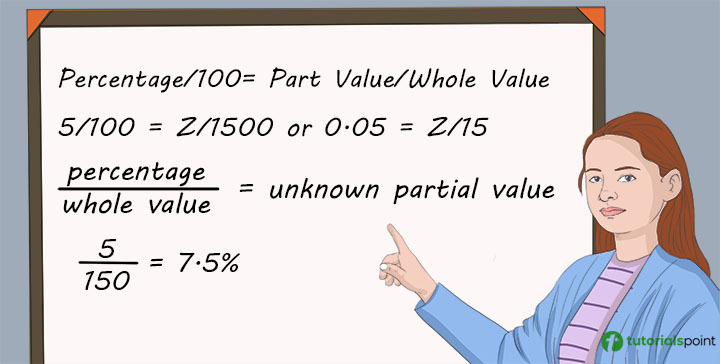What if you are given a partial value and a percentage used to express it, and you need to find the whole value it belongs to? You can use the same equation as in the method above −

P/100 = Part Value/Whole Value.


For example, if 5% of the students in a college are equal to 20 students, what would the total class strength be? It would be 5/100=20/Y or 20/0.05, which yields 400 students.

Alternatively, it can be expressed with the statement X of Y Equals Z, or 5% of Y equals 20, where Y is found to be 400.## Method 5: Finding One Percentage from Another Percentage

If we are given a partial value and want to find the percentage of the remaining portion percentage, without knowing the whole - there is a quick and easy way to do so. During a catering event, 42 pies were eaten, and 10 went to waste. How do we find the percentage of the consumed pies out of the total?

First, find the total by adding up the eaten and wasted pies, i.e., 52 pies in total. Then create a fraction using the eaten pies and the total – in other words, the part value and the whole value ratio, which is 42/52. You can multiply the fraction by 100 or divide the numerator by the denominator to get the decimal. i.e., 0.81, and multiply the decimal value by 100. So, 81% of the pies were eaten, whereas 19% went to waste.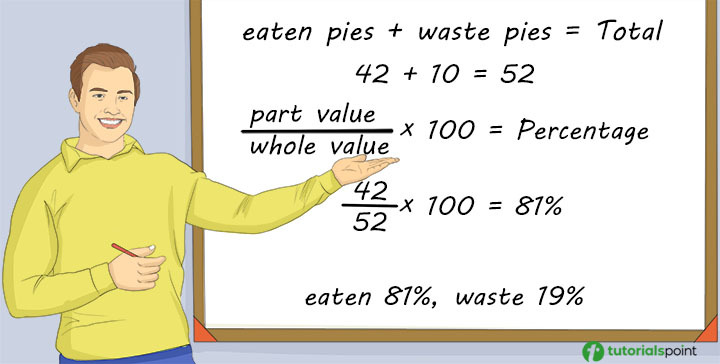## Conclusion

With these tips in your arsenal, percentage problems will be a thing of the past! It's time to go out and show off your new mental maths skills!

Updated on: 04-May-2023

51 Views